Home > CC4 > Chapter A > Lesson A.1.5 > ProblemA-56

A-56.

A-56. Calculate the following values. Homework Help ✎

1. If y = 2 + 4.3x, what is the value of y when x = −6?

2. If g(x) = (x − 3)2, what is the value of g(−9)?

3. If C(x) = x − 2, what is the value of x when C(x) = 3.5?

4. If y = 5x2 − 4, what is the value of y when x = −2?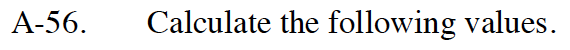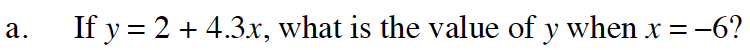Substitute −6 in for x.
y = 2 + 4.3( −6 )

Multiply and simplify.

y = 2 + (−25.8)

y = −23.8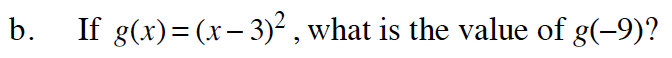Follow the steps in part (a).

Remember to use the correct order of operations.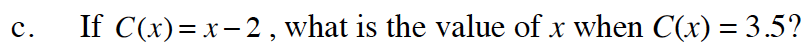Begin by substituting 3.5 for x in the equation.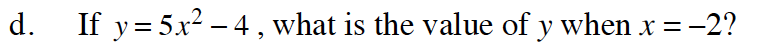Rewrite the equation as y = 5(−2)² − 4.

16